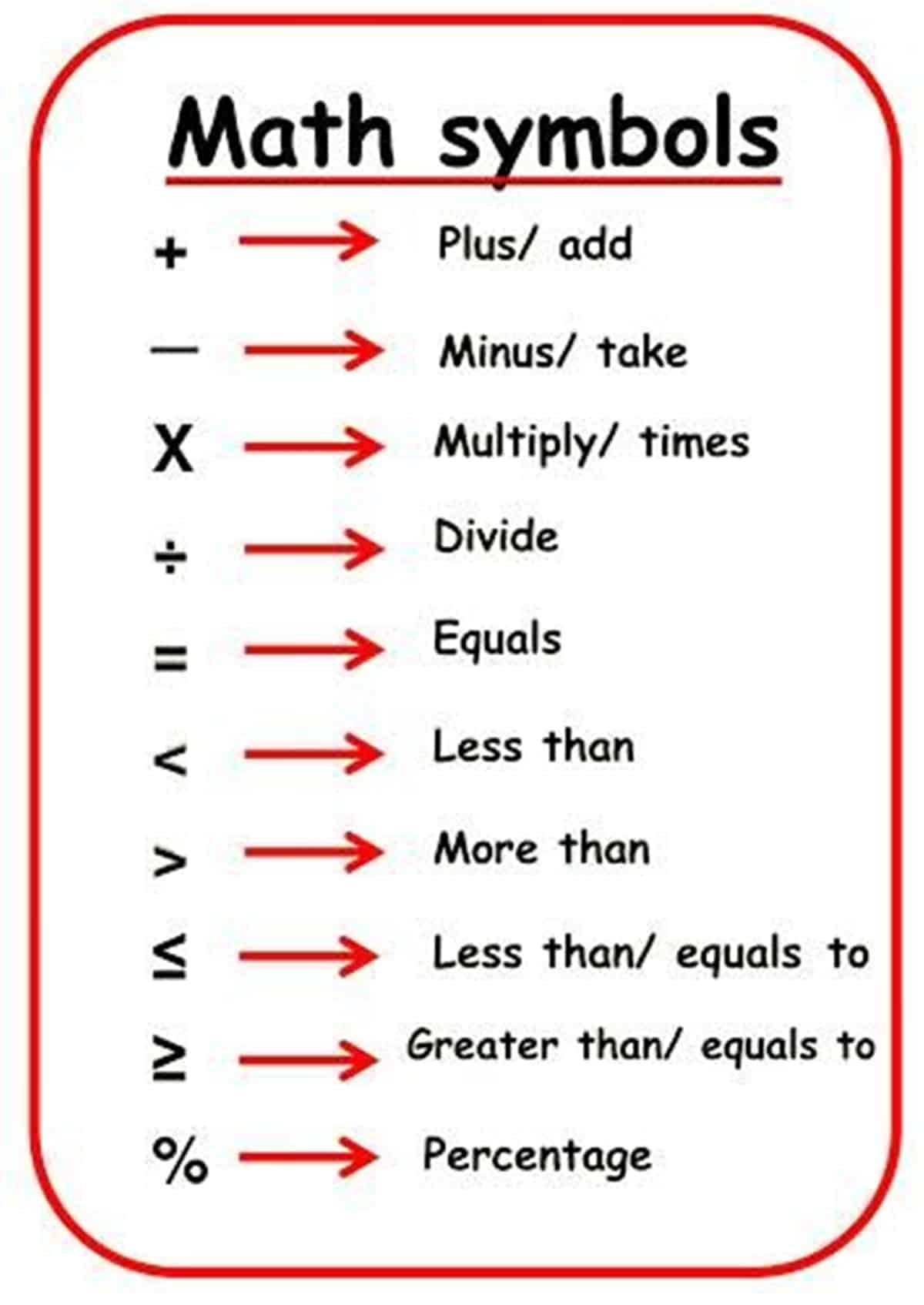# Math symbols in English

Sharing is caring!

Mathematics is used to communicate information about a wide range of different subjects. Let’s learn some math symbols in English.

Contents

## Math Symbols in English

Math Vocabulary

• “=” – equals sign :

5 = 2+3:  5 is equal to 2+3

• “≠”  not equal sign

5 ≠ 4 : 5 is not equal to 4

• “≈” – approximately equal

(0.01) ≈ 0.01,

x ≈ y means x is approximately equal to y

• “>” – greater than

5 > 4 : 5 is greater than 4

• “<” – less than

4 < 5: 4 is less than 5

• “≥” – greater than or equal to

5 ≥ 4, x ≥ y means x is greater than or equal to y

• “≤” – less than or equal to

x ≤ y means x is less than or equal to y

## Math Symbols | Images• “+” – plus: 1 + 1 = 2
• “−” – minus : 2 − 1 = 1
• “×” multiplication :2 × 3 = 6
• “⋅” – dotmultiplication: 2 ⋅ 3 = 6
• “÷” – divide : 6 ÷ 2 = 3
• “√a” – square root: √a ⋅ √a  = a
• “%” – percent: 1% = 1/10010% × 30 = 3
• “‰” – per-mille: 1‰ = 1/1000 = 0.1%10‰ × 30 = 0.3## Author

•San nuam

Saturday 7th of September 2019

like it 👍👍👍👍

Jean benedicto zúñiga

Wednesday 25th of October 2017

Very good and useful